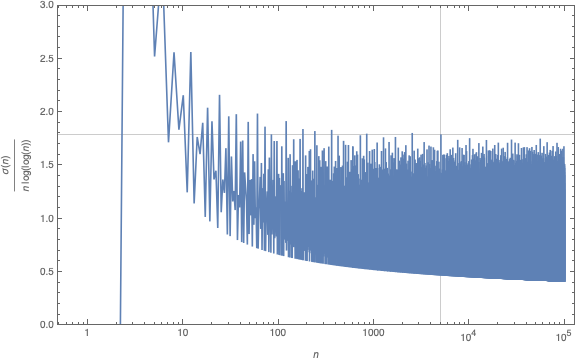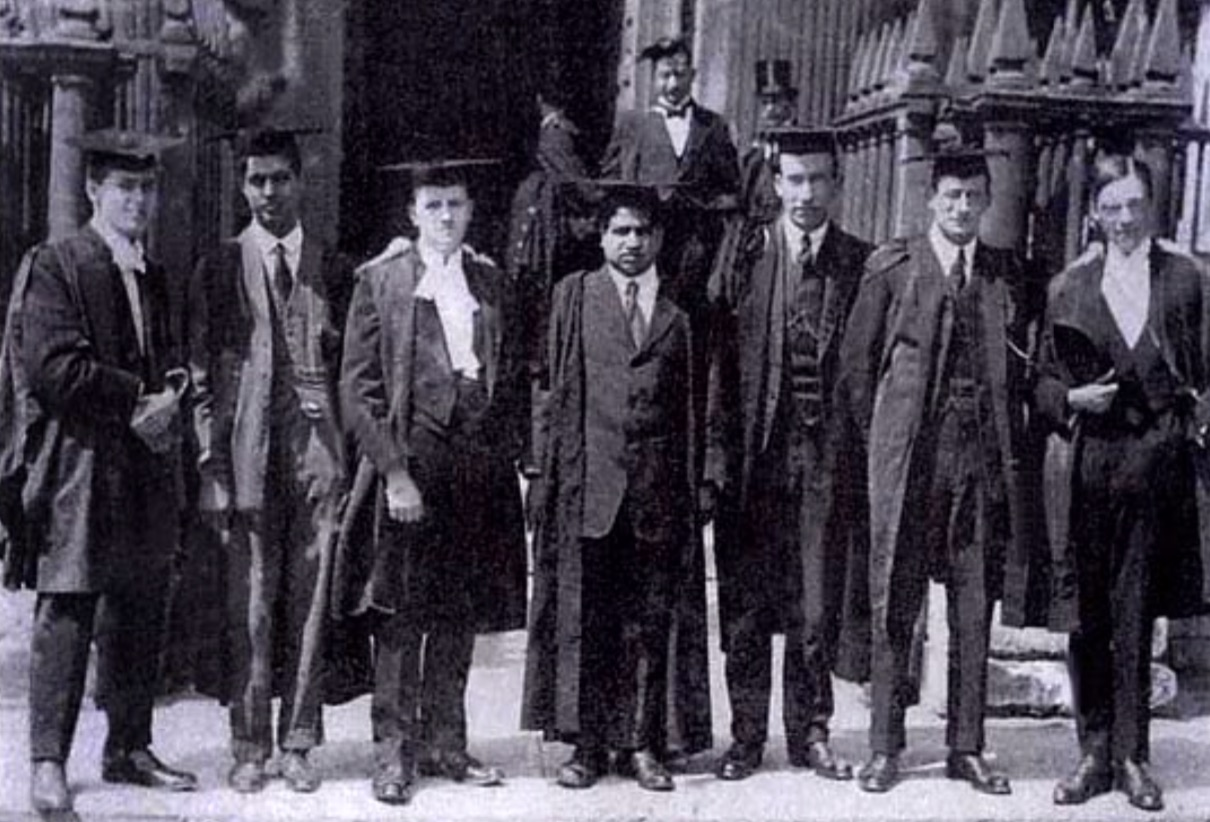# Tag: Riemann hypothesis

In recent months, my primary focus was on teaching and family matters, so I make advantage of this Christmas break to catch up with some of the things I’ve missed.

Peter Woit’s blog alerted me to the existence of the (virtual) Lake Como-conference, end of september: Unifying themes in Geometry.

In Corona times, virtual conferences seem to sprout up out of nowhere, everywhere (zero costs), giving us an inflation of YouTubeD talks. I’m always grateful to the organisers of such events to provide the slides of the talks separately, as the generic YouTubeD-talk consists merely in reading off the slides.

Allow me to point you to one of the rare exceptions to this rule.

When I downloaded the slides of Alain Connes’ talk at the conference From noncommutative geometry to the tropical geometry of the scaling site I just saw a collage of graphics from his endless stream of papers with Katia Consani, and slides I’d seen before watching several of his YouTubeD-talks in recent years.

Boy, am I glad I gave Alain 5 minutes to convince me this talk was different.

For the better part of his talk, Alain didn’t just read off the slides, but rather tried to explain the thought processes that led him and Katia to move on from the results on this slide to those on the next one.

If you’re pressed for time, perhaps you might join in at 49.34 into the talk, when he acknowledges the previous (tropical) approach ran out of steam as they were unable to define any $H^1$ properly, and how this led them to ‘absolute’ algebraic geometry, meaning over the sphere spectrum $\mathbb{S}$.

Sadly, for some reason Alain didn’t manage to get his final two slides on screen. So, in this case, the slides actually add value to the talk…

Yesterday, there was an interesting post by John Baez at the n-category cafe: The Riemann Hypothesis Says 5040 is the Last.

The 5040 in the title refers to the largest known counterexample to a bound for the sum-of-divisors function
$\sigma(n) = \sum_{d | n} d = n \sum_{d | n} \frac{1}{d}$

In 1983, the french mathematician Guy Robin proved that the Riemann hypothesis is equivalent to
$\frac{\sigma(n)}{n~log(log(n))} < e^{\gamma} = 1.78107...$ when $n > 5040$.

The other known counterexamples to this bound are the numbers 3,4,5,6,8,9,10,12,16,18,20,24,30,36,48,60,72,84,120,180,240,360,720,840,2520.In Baez’ post there is a nice graph of this function made by Nicolas Tessore, with 5040 indicated with a grey line towards the right and the other counterexamples jumping over the bound 1.78107…Robin’s theorem has a remarkable history, starting in 1915 with good old Ramanujan writing a part of this thesis on “highly composite numbers” (numbers divisible by high powers of primes).

His PhD. adviser Hardy liked his result but called them “in the backwaters of mathematics” and most of it was not published at the time of Ramanujan’s degree ceremony in 1916, due to paper shortage in WW1.When Ramanujan’s paper “Highly Composite Numbers” was first published in 1988 in ‘The lost notebook and other unpublished papers’ it became clear that Ramanujan had already part of Robin’s theorem.

Ramanujan states that if the Riemann hypothesis is true, then for $n_0$ large enough we must have for all $n > n_0$ that
$\frac{\sigma(n)}{n~log(log(n))} < e^{\gamma} = 1.78107...$ When Jean-Louis Nicolas, Robin's PhD. adviser, read Ramanujan's lost notes he noticed that there was a sign error in Ramanujan's formula which prevented him from seeing Robin's theorem.

Nicolas: “Soon after discovering the hidden part, I read it and saw the difference between Ramanujan’s result and Robin’s one. Of course, I would have bet that the error was in Robin’s paper, but after recalculating it several times and asking Robin to check, it turned out that there was an error of sign in what Ramanujan had written.”

If you are interested in the full story, read the paper by Jean-Louis Nicolas and Jonathan Sondow: Ramanujan, Robin, Highly Composite Numbers, and the Riemann Hypothesis.

What’s the latest on Robin’s inequality? An arXiv-search for Robin’s inequality shows a flurry of activity.

For starters, it has been verified for all numbers smaller that $10^{10^{13}}$…

It has been verified, unconditionally, for certain classes of numbers:

• all odd integers $> 9$
• all numbers not divisible by a 25-th power of a prime

Rings a bell? Here’s another hint:

According to Xiaolong Wu in A better method than t-free for Robin’s hypothesis one can replace the condition of ‘not divisible by an N-th power of a prime’ by ‘not divisible by an N-th power of 2’.

Further, he claims to have an (as yet unpublished) argument that Robin’s inequality holds for all numbers not divisible by $2^{42}$.

So, where should we look for counterexamples to the Riemann hypothesis?

What about the orders of huge simple groups?

The order of the Monster group is too small to be a counterexample (yet, it is divisible by $2^{46}$).

‘Gabriel’s topos’ (see here) is the conjectural, but still elusive topos from which the validity of the Riemann hypothesis would follow.

It is the latest attempt in Alain Connes’ 20 year long quest to tackle the RH (before, he tried the tools of noncommutative geometry and later those offered by the field with one element).

For the last 5 years he hopes that topos theory might provide the missing ingredient. Together with Katia Consani he introduced and studied the geometry of the Arithmetic site, and later the geometry of the scaling site.

If you look at the points of these toposes you get horribly complicated ‘non-commutative’ spaces, such as the finite adele classes $\mathbb{Q}^*_+ \backslash \mathbb{A}^f_{\mathbb{Q}} / \widehat{\mathbb{Z}}^{\ast}$ (in case of the arithmetic site) and the full adele classes $\mathbb{Q}^*_+ \backslash \mathbb{A}_{\mathbb{Q}} / \widehat{\mathbb{Z}}^{\ast}$ (for the scaling site).

In Vienna, Connes gave a nice introduction to the arithmetic site in two lectures. The first part of the talk below also gives an historic overview of his work on the RH

The second lecture can be watched here.

However, not everyone is as optimistic about the topos-approach as he seems to be. Here’s an insightful answer on MathOverflow by Will Sawin to the question “What is precisely still missing in Connes’ approach to RH?”.

Other interesting MathOverflow threads related to the RH-approach via the field with one element are Approaches to Riemann hypothesis using methods outside number theory and Riemann hypothesis via absolute geometry.

About a month ago, from May 10th till 14th Alain Connes gave a series of lectures at Ohio State University with title “The Riemann-Roch strategy, quantizing the Scaling Site”.

The accompanying paper has now been arXived: The Riemann-Roch strategy, Complex lift of the Scaling Site (joint with K. Consani).

Especially interesting is section 2 “The geometry behind the zeros of $\zeta$” in which they explain how looking at the zeros locus inevitably leads to the space of adele classes and why one has to study this space with the tools from noncommutative geometry.

Perhaps further developments will be disclosed in a few weeks time when Connes is one of the speakers at Toposes in Como.